# http www comp nus edu sgcs 1010 UNIT

• Slides: 30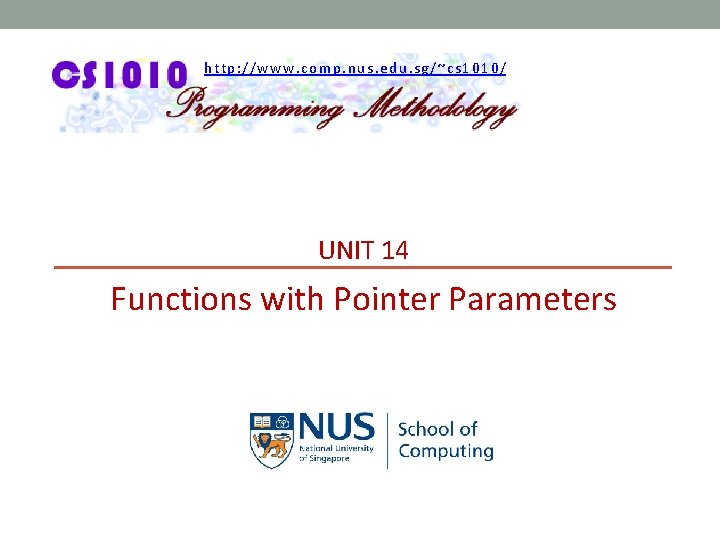http: //www. comp. nus. edu. sg/~cs 1010/ UNIT 14 Functions with Pointer Parameters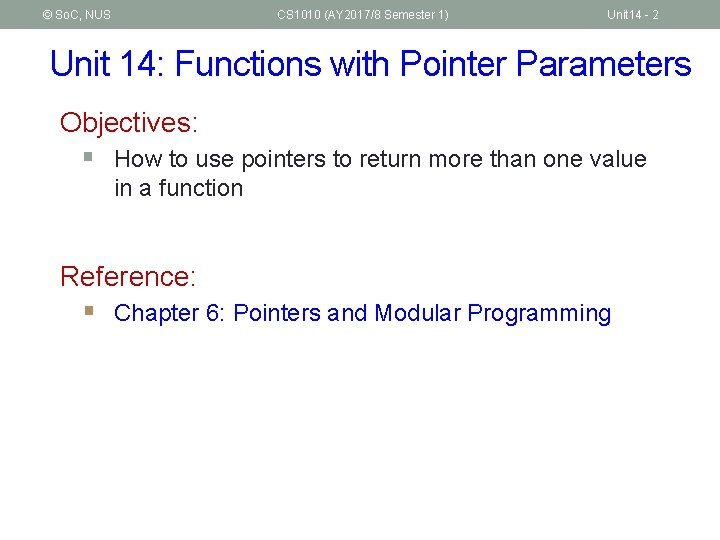© So. C, NUS CS 1010 (AY 2017/8 Semester 1) Unit 14 - 2 Unit 14: Functions with Pointer Parameters Objectives: § How to use pointers to return more than one value in a function Reference: § Chapter 6: Pointers and Modular Programming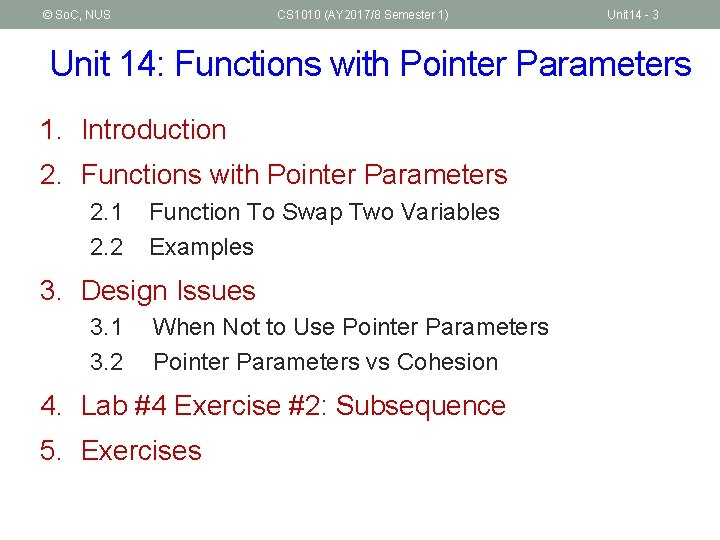© So. C, NUS CS 1010 (AY 2017/8 Semester 1) Unit 14 - 3 Unit 14: Functions with Pointer Parameters 1. Introduction 2. Functions with Pointer Parameters 2. 1 2. 2 Function To Swap Two Variables Examples 3. Design Issues 3. 1 3. 2 When Not to Use Pointer Parameters vs Cohesion 4. Lab #4 Exercise #2: Subsequence 5. Exercises© So. C, NUS CS 1010 (AY 2017/8 Semester 1) Unit 14 - 4 1. Introduction (1/4) § In Unit #5, we learned that a function may return a value, or it may not return any value at all (void function) § Is it possible for a function to return 2 or more values? § Does the following function f(n) return both 2 n and 3 n? int f(int n) { return 2 * n; return 3 * n; } § No, f(n) returns only 2 n. § Once a return statement is executed, the function terminates immediately.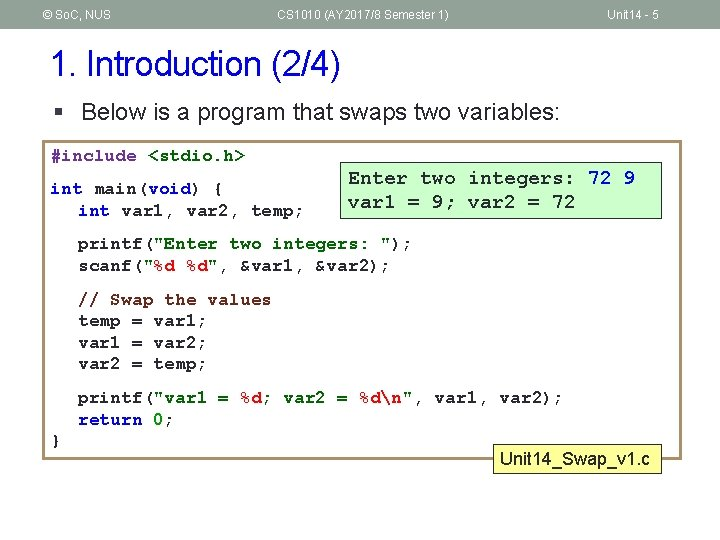© So. C, NUS CS 1010 (AY 2017/8 Semester 1) Unit 14 - 5 1. Introduction (2/4) § Below is a program that swaps two variables: #include <stdio. h> int main(void) { int var 1, var 2, temp; Enter two integers: 72 9 var 1 = 9; var 2 = 72 printf("Enter two integers: "); scanf("%d %d", &var 1, &var 2); // Swap the values temp = var 1; var 1 = var 2; var 2 = temp; printf("var 1 = %d; var 2 = %dn", var 1, var 2); return 0; } Unit 14_Swap_v 1. c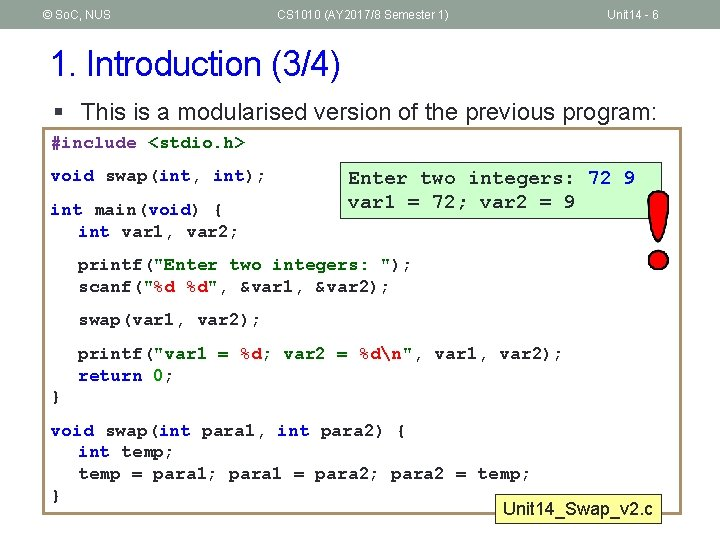© So. C, NUS CS 1010 (AY 2017/8 Semester 1) Unit 14 - 6 1. Introduction (3/4) § This is a modularised version of the previous program: #include <stdio. h> void swap(int, int); int main(void) { int var 1, var 2; Enter two integers: 72 9 var 1 = 72; var 2 = 9 printf("Enter two integers: "); scanf("%d %d", &var 1, &var 2); swap(var 1, var 2); printf("var 1 = %d; var 2 = %dn", var 1, var 2); return 0; } void swap(int para 1, int para 2) { int temp; temp = para 1; para 1 = para 2; para 2 = temp; } Unit 14_Swap_v 2. c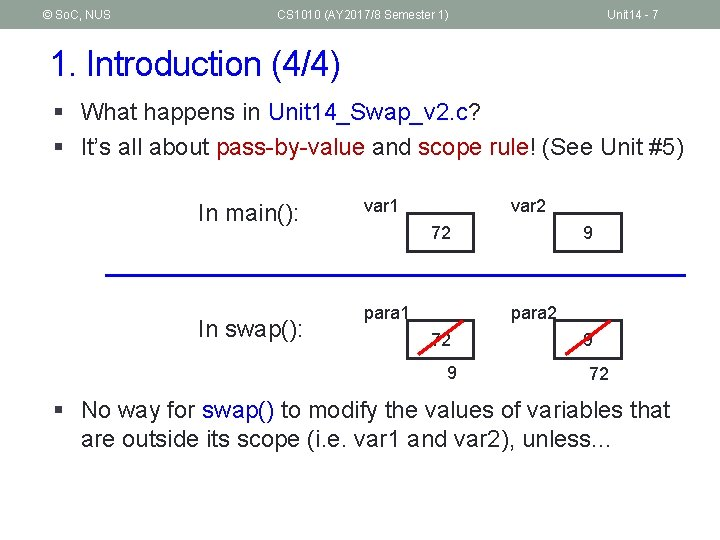© So. C, NUS CS 1010 (AY 2017/8 Semester 1) Unit 14 - 7 1. Introduction (4/4) § What happens in Unit 14_Swap_v 2. c? § It’s all about pass-by-value and scope rule! (See Unit #5) In main(): In swap(): var 1 var 2 72 para 1 9 para 2 72 9 9 72 § No way for swap() to modify the values of variables that are outside its scope (i. e. var 1 and var 2), unless. . .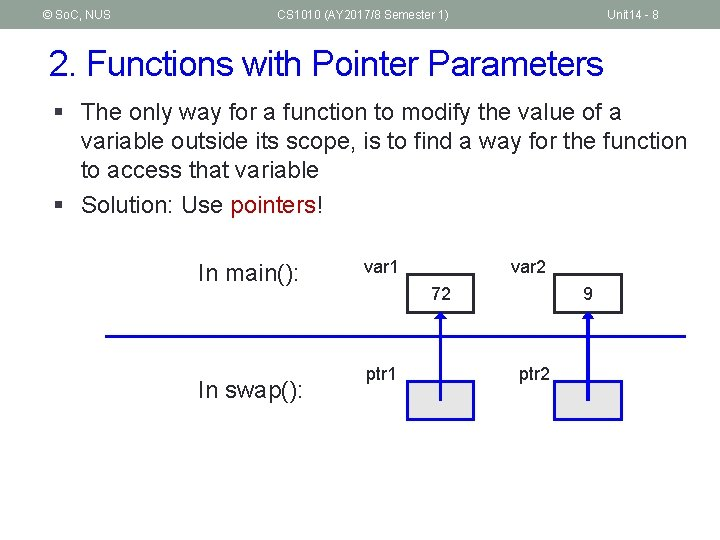© So. C, NUS CS 1010 (AY 2017/8 Semester 1) Unit 14 - 8 2. Functions with Pointer Parameters § The only way for a function to modify the value of a variable outside its scope, is to find a way for the function to access that variable § Solution: Use pointers! In main(): In swap(): var 1 var 2 72 ptr 1 9 ptr 2© So. C, NUS CS 1010 (AY 2017/8 Semester 1) Unit 14 - 9 2. 1 Function to Swap Two Variables 9 § Here’s the solution In main(): var 1 In swap(): ptr 1 72 var 2 72 9 #include <stdio. h> void swap(int *, int *); ptr 2 int main(void) { int var 1, var 2; printf("Enter two integers: "); scanf("%d %d", &var 1, &var 2); swap(&var 1, &var 2); printf("var 1 = %d; var 2 = %dn", var 1, var 2); return 0; } void swap(int *ptr 1, int *ptr 2) { int temp; temp = *ptr 1; *ptr 1 = *ptr 2; *ptr 2 = temp; } Unit 14_Swap_v 3. c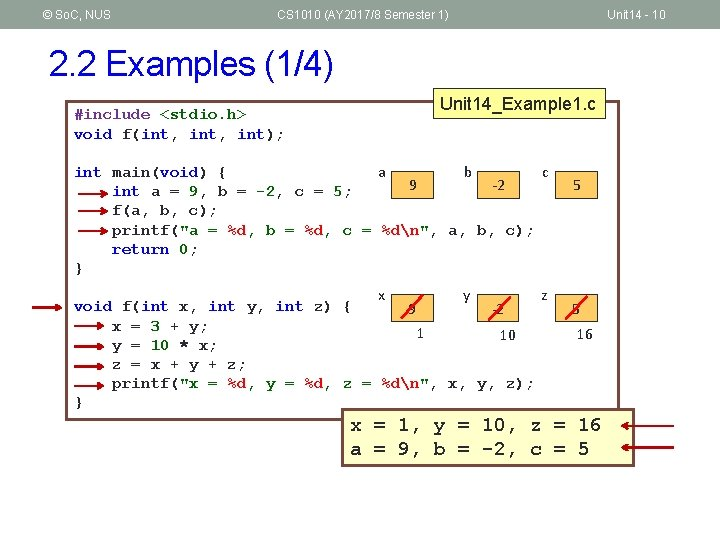© So. C, NUS CS 1010 (AY 2017/8 Semester 1) Unit 14 - 10 2. 2 Examples (1/4) #include <stdio. h> void f(int, int); Unit 14_Example 1. c a b c int main(void) { 9 -2 int a = 9, b = -2, c = 5; f(a, b, c); printf("a = %d, b = %d, c = %dn", a, b, c); return 0; } x y z void f(int x, int y, int z) { 9 -2 x = 3 + y; 1 10 y = 10 * x; z = x + y + z; printf("x = %d, y = %d, z = %dn", x, y, z); } 5 5 16 x = 1, y = 10, z = 16 a = 9, b = -2, c = 5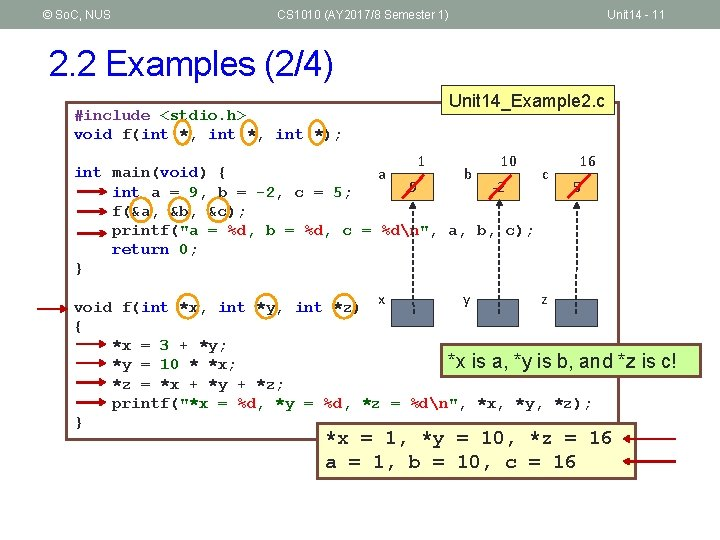© So. C, NUS CS 1010 (AY 2017/8 Semester 1) Unit 14 - 11 2. 2 Examples (2/4) #include <stdio. h> void f(int *, int *); Unit 14_Example 2. c 1 10 int main(void) { a b c 9 -2 int a = 9, b = -2, c = 5; f(&a, &b, &c); printf("a = %d, b = %d, c = %dn", a, b, c); return 0; } 16 5 y z void f(int *x, int *y, int *z) x { *x = 3 + *y; *x is a, *y is b, and *y = 10 * *x; *z = *x + *y + *z; printf("*x = %d, *y = %d, *z = %dn", *x, *y, *z); } *x = 1, *y = 10, *z = 16 a = 1, b = 10, c = 16 *z is c!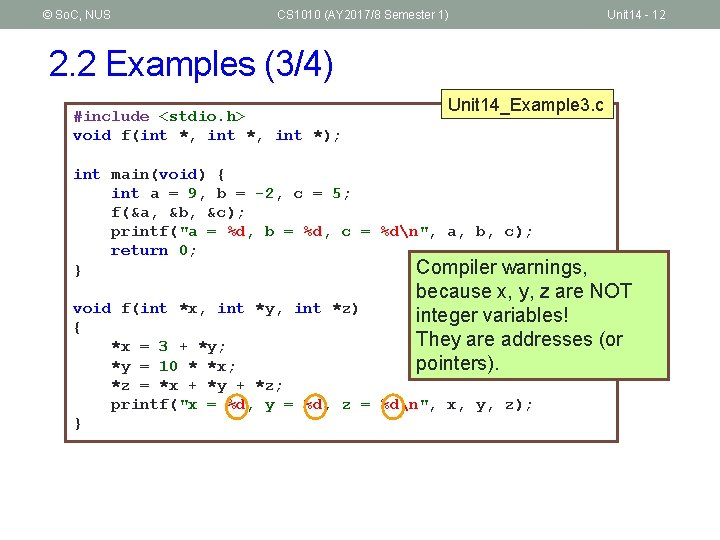© So. C, NUS CS 1010 (AY 2017/8 Semester 1) Unit 14 - 12 2. 2 Examples (3/4) #include <stdio. h> void f(int *, int *); Unit 14_Example 3. c int main(void) { int a = 9, b = -2, c = 5; f(&a, &b, &c); printf("a = %d, b = %d, c = %dn", a, b, c); return 0; Compiler warnings, } because x, y, z are NOT integer variables! They are addresses (or pointers). void f(int *x, int *y, int *z) { *x = 3 + *y; *y = 10 * *x; *z = *x + *y + *z; printf("x = %d, y = %d, z = %dn", x, y, z); }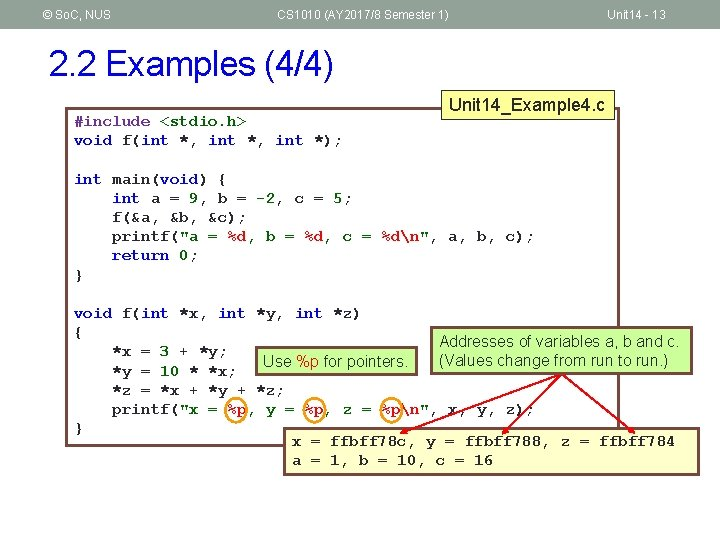© So. C, NUS CS 1010 (AY 2017/8 Semester 1) Unit 14 - 13 2. 2 Examples (4/4) #include <stdio. h> void f(int *, int *); Unit 14_Example 4. c int main(void) { int a = 9, b = -2, c = 5; f(&a, &b, &c); printf("a = %d, b = %d, c = %dn", a, b, c); return 0; } void f(int *x, int *y, int *z) { Addresses of variables a, b and c. *x = 3 + *y; (Values change from run to run. ) Use %p for pointers. *y = 10 * *x; *z = *x + *y + *z; printf("x = %p, y = %p, z = %pn", x, y, z); } x = ffbff 78 c, y = ffbff 788, z = ffbff 784 a = 1, b = 10, c = 16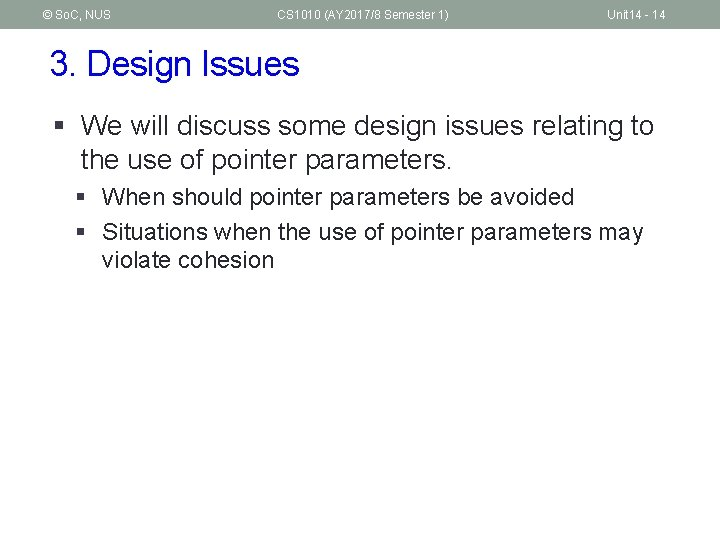© So. C, NUS CS 1010 (AY 2017/8 Semester 1) Unit 14 - 14 3. Design Issues § We will discuss some design issues relating to the use of pointer parameters. § When should pointer parameters be avoided § Situations when the use of pointer parameters may violate cohesion© So. C, NUS CS 1010 (AY 2017/8 Semester 1) Unit 14 - 15 3. 1 When Not to Use Pointer Parameters § Both programs are correct, but which is preferred? Why? (A) Unit 14_Print_v 1. c int main(void) { int num 1 = 1, num 2 = 2; print_values(num 1, num 2); return 0; } void print_values(int n 1, int n 2) { printf("Values: %d and %d", n 1, n 2); } (B) int main(void) { int num 1 = 1, num 2 = 2; print_values(&num 1, &num 2); return 0; } void print_values(int *n 1, int *n 2) { printf("Values: %d and %d", *n 1, *n 2); } Unit 14_Print_v 2. c § (B) does not allow calls like print_values(3, 4), print_values(a+b, c*d), etc. , whereas (A) does. § Use pointer parameters only if absolutely necessary.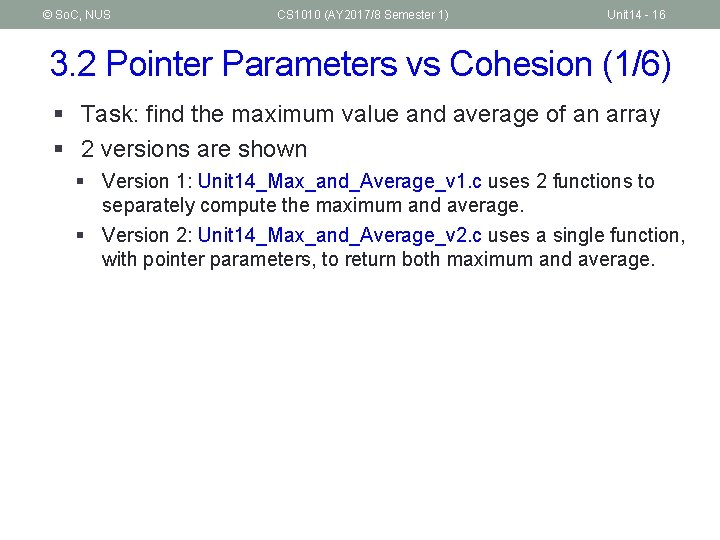© So. C, NUS CS 1010 (AY 2017/8 Semester 1) Unit 14 - 16 3. 2 Pointer Parameters vs Cohesion (1/6) § Task: find the maximum value and average of an array § 2 versions are shown § Version 1: Unit 14_Max_and_Average_v 1. c uses 2 functions to separately compute the maximum and average. § Version 2: Unit 14_Max_and_Average_v 2. c uses a single function, with pointer parameters, to return both maximum and average.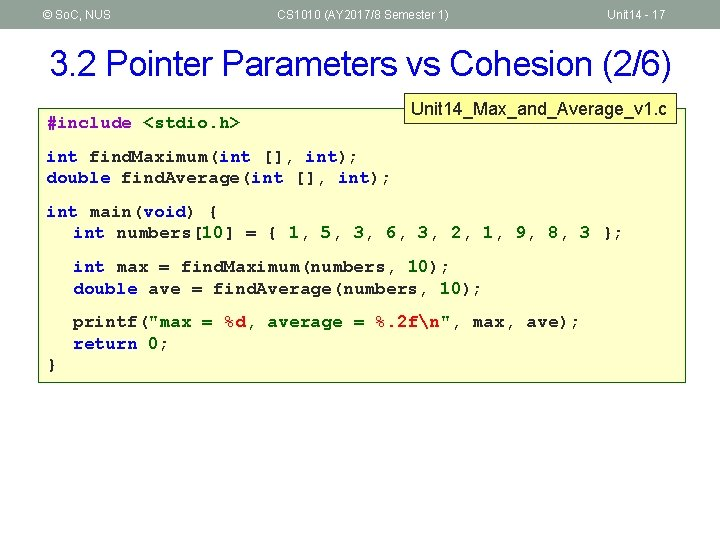© So. C, NUS CS 1010 (AY 2017/8 Semester 1) Unit 14 - 17 3. 2 Pointer Parameters vs Cohesion (2/6) #include <stdio. h> Unit 14_Max_and_Average_v 1. c int find. Maximum(int [], int); double find. Average(int [], int); int main(void) { int numbers = { 1, 5, 3, 6, 3, 2, 1, 9, 8, 3 }; int max = find. Maximum(numbers, 10); double ave = find. Average(numbers, 10); printf("max = %d, average = %. 2 fn", max, ave); return 0; }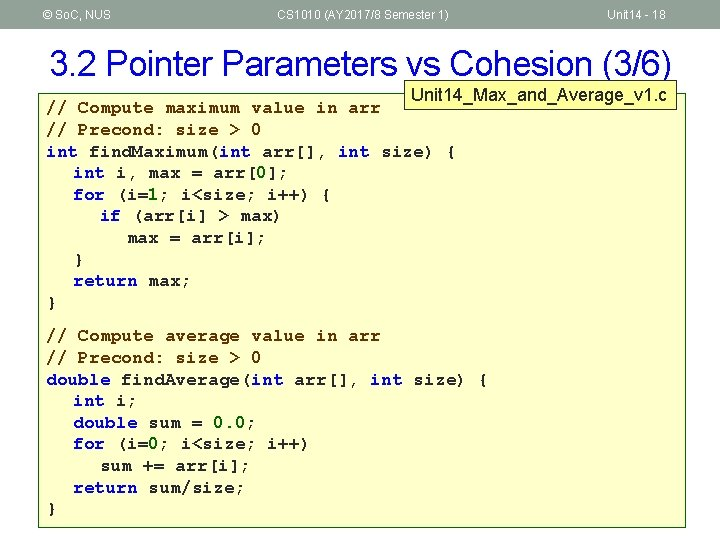© So. C, NUS CS 1010 (AY 2017/8 Semester 1) Unit 14 - 18 3. 2 Pointer Parameters vs Cohesion (3/6) Unit 14_Max_and_Average_v 1. c // Compute maximum value in arr // Precond: size > 0 int find. Maximum(int arr[], int size) { int i, max = arr; for (i=1; i<size; i++) { if (arr[i] > max) max = arr[i]; } return max; } // Compute average value in arr // Precond: size > 0 double find. Average(int arr[], int size) { int i; double sum = 0. 0; for (i=0; i<size; i++) sum += arr[i]; return sum/size; }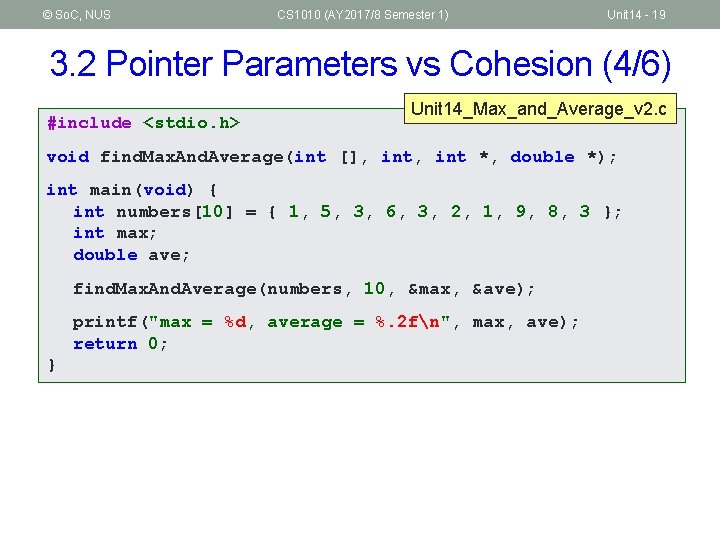© So. C, NUS CS 1010 (AY 2017/8 Semester 1) Unit 14 - 19 3. 2 Pointer Parameters vs Cohesion (4/6) #include <stdio. h> Unit 14_Max_and_Average_v 2. c void find. Max. And. Average(int [], int *, double *); int main(void) { int numbers = { 1, 5, 3, 6, 3, 2, 1, 9, 8, 3 }; int max; double ave; find. Max. And. Average(numbers, 10, &max, &ave); printf("max = %d, average = %. 2 fn", max, ave); return 0; }© So. C, NUS CS 1010 (AY 2017/8 Semester 1) Unit 14 - 20 3. 2 Pointer Parameters vs Cohesion (5/6) // Compute maximum value and average value in arr // Precond: size > 0 void find. Max. And. Average(int arr[], int size, int *max_ptr, double *ave_ptr) { int i; double sum = 0. 0; *max_ptr = arr; for (i=0; i<size; i++) { if (arr[i] > *max_ptr) { *max_ptr = arr[i]; } sum += arr[i]; } *ave_ptr = sum/size; } Unit 14_Max_and_Average_v 2. c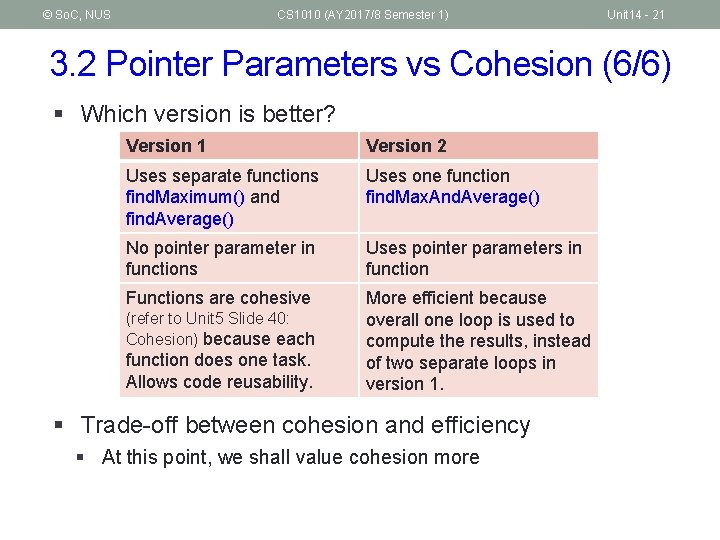© So. C, NUS CS 1010 (AY 2017/8 Semester 1) Unit 14 - 21 3. 2 Pointer Parameters vs Cohesion (6/6) § Which version is better? Version 1 Version 2 Uses separate functions find. Maximum() and find. Average() Uses one function find. Max. And. Average() No pointer parameter in functions Uses pointer parameters in function Functions are cohesive More efficient because overall one loop is used to compute the results, instead of two separate loops in version 1. (refer to Unit 5 Slide 40: Cohesion) because each function does one task. Allows code reusability. § Trade-off between cohesion and efficiency § At this point, we shall value cohesion more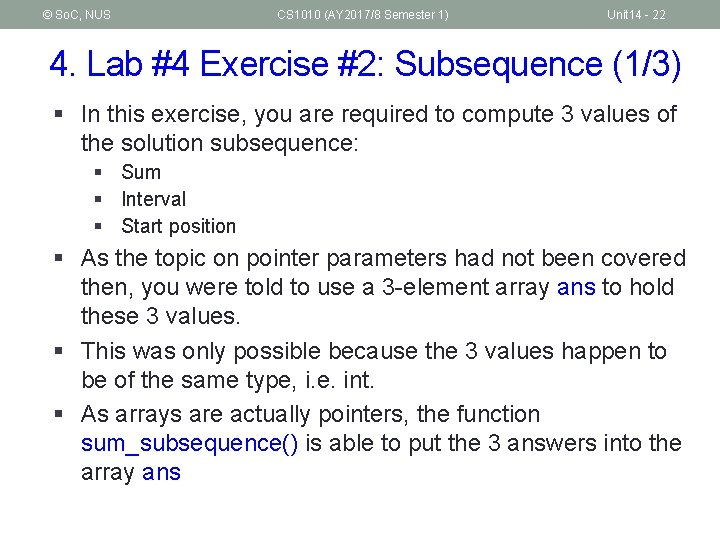© So. C, NUS CS 1010 (AY 2017/8 Semester 1) Unit 14 - 22 4. Lab #4 Exercise #2: Subsequence (1/3) § In this exercise, you are required to compute 3 values of the solution subsequence: § Sum § Interval § Start position § As the topic on pointer parameters had not been covered then, you were told to use a 3 -element array ans to hold these 3 values. § This was only possible because the 3 values happen to be of the same type, i. e. int. § As arrays are actually pointers, the function sum_subsequence() is able to put the 3 answers into the array ans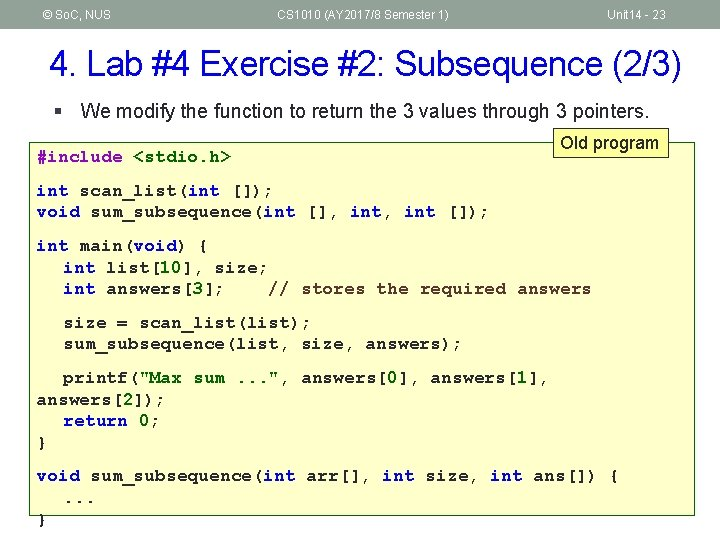© So. C, NUS CS 1010 (AY 2017/8 Semester 1) Unit 14 - 23 4. Lab #4 Exercise #2: Subsequence (2/3) § We modify the function to return the 3 values through 3 pointers. #include <stdio. h> Old program int scan_list(int []); void sum_subsequence(int [], int []); int main(void) { int list, size; int answers; // stores the required answers size = scan_list(list); sum_subsequence(list, size, answers); printf("Max sum. . . ", answers, answers, answers); return 0; } void sum_subsequence(int arr[], int size, int ans[]) {. . . }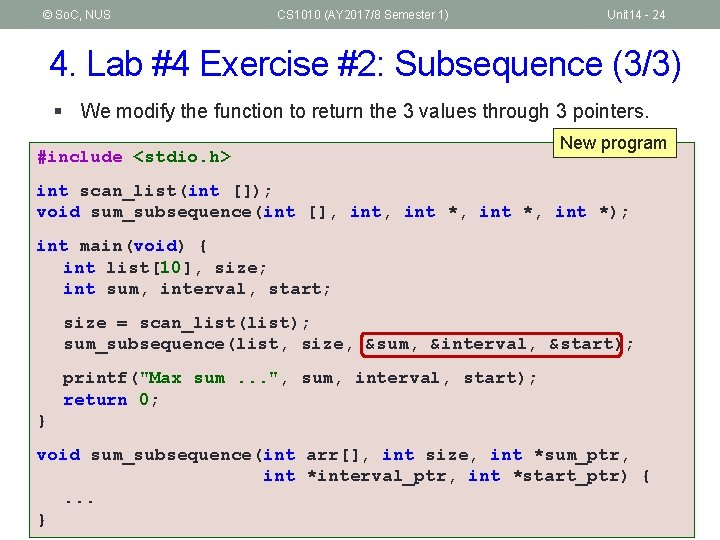© So. C, NUS CS 1010 (AY 2017/8 Semester 1) Unit 14 - 24 4. Lab #4 Exercise #2: Subsequence (3/3) § We modify the function to return the 3 values through 3 pointers. #include <stdio. h> New program int scan_list(int []); void sum_subsequence(int [], int *, int *); int main(void) { int list, size; int sum, interval, start; size = scan_list(list); sum_subsequence(list, size, &sum, &interval, &start); printf("Max sum. . . ", sum, interval, start); return 0; } void sum_subsequence(int arr[], int size, int *sum_ptr, int *interval_ptr, int *start_ptr) {. . . }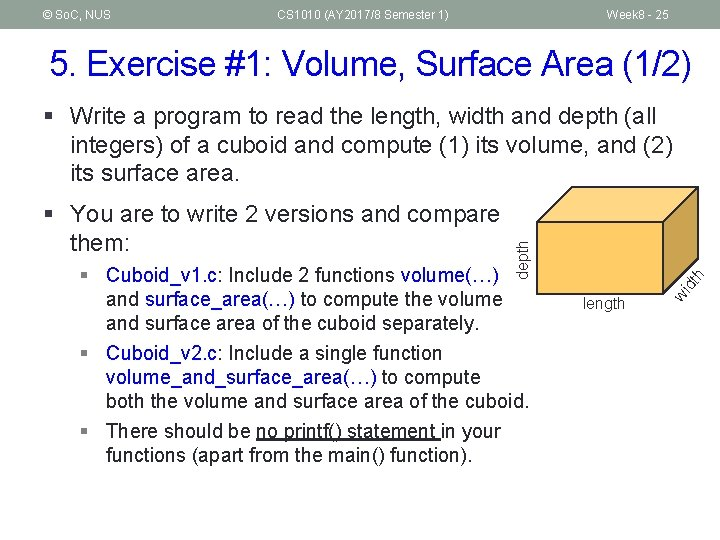© So. C, NUS CS 1010 (AY 2017/8 Semester 1) Week 8 - 25 5. Exercise #1: Volume, Surface Area (1/2) h dt § Cuboid_v 1. c: Include 2 functions volume(…) and surface_area(…) to compute the volume and surface area of the cuboid separately. § Cuboid_v 2. c: Include a single function volume_and_surface_area(…) to compute both the volume and surface area of the cuboid. § There should be no printf() statement in your functions (apart from the main() function). length wi § You are to write 2 versions and compare them: depth § Write a program to read the length, width and depth (all integers) of a cuboid and compute (1) its volume, and (2) its surface area.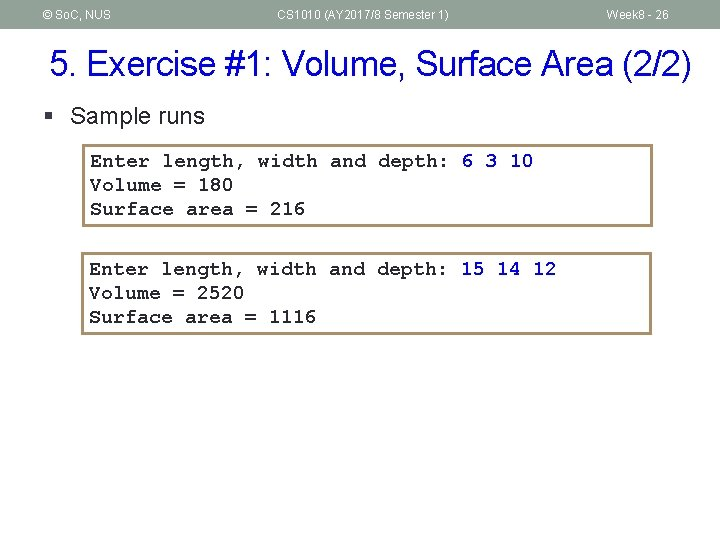© So. C, NUS CS 1010 (AY 2017/8 Semester 1) Week 8 - 26 5. Exercise #1: Volume, Surface Area (2/2) § Sample runs Enter length, width and depth: 6 3 10 Volume = 180 Surface area = 216 Enter length, width and depth: 15 14 12 Volume = 2520 Surface area = 1116© So. C, NUS CS 1010 (AY 2017/8 Semester 1) Week 8 - 27 5. Exercise #2: Triangle Centroid (1/2) § In a triangle, a median is a line that connects a vertex to the midpoint of its opposite side. (eg: blue dotted lines) § The intersection of the 3 medians is called the centroid. (eg: point G) P (x 1, y 1) G Q (x 2, y 2) R (x 3, y 3) § Write a program triangle. Centroid. c to read in the coordinates (of type float) of 3 vertices of a triangle and compute the coordinates of its centroid. § Your program should have a function centroid(…). § There should be no printf() statement in this centroid() function. § This exercise is mounted on Code. Crunch.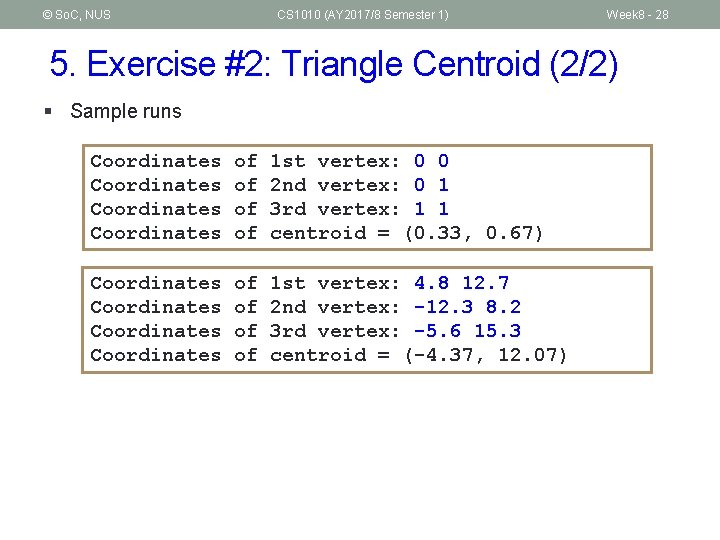© So. C, NUS CS 1010 (AY 2017/8 Semester 1) Week 8 - 28 5. Exercise #2: Triangle Centroid (2/2) § Sample runs Coordinates of of 1 st vertex: 0 0 2 nd vertex: 0 1 3 rd vertex: 1 1 centroid = (0. 33, 0. 67) Coordinates of of 1 st vertex: 4. 8 12. 7 2 nd vertex: -12. 3 8. 2 3 rd vertex: -5. 6 15. 3 centroid = (-4. 37, 12. 07)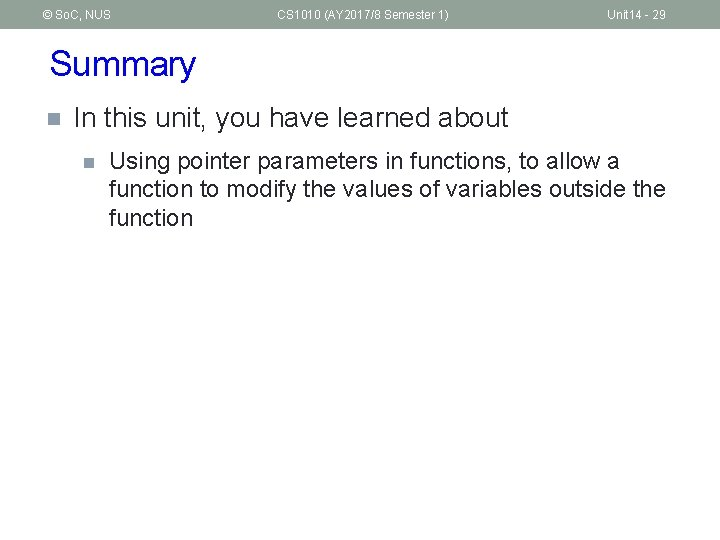© So. C, NUS CS 1010 (AY 2017/8 Semester 1) Unit 14 - 29 Summary n In this unit, you have learned about n Using pointer parameters in functions, to allow a function to modify the values of variables outside the function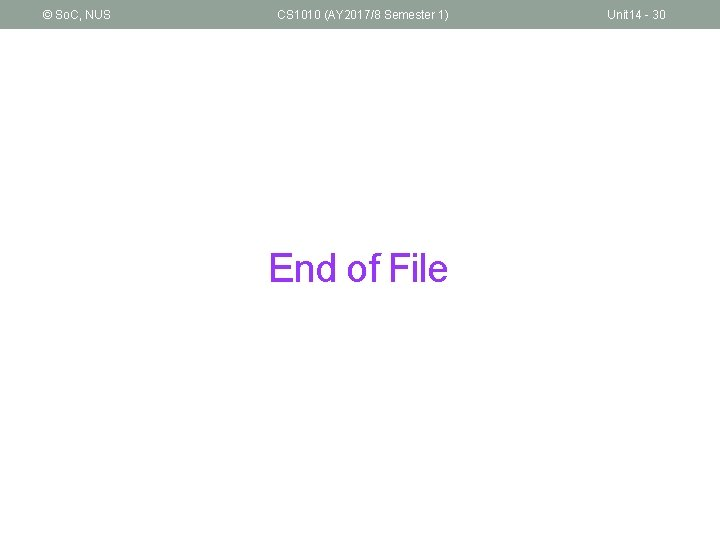© So. C, NUS CS 1010 (AY 2017/8 Semester 1) End of File Unit 14 - 30